# The Four Types of Estimable Functions

## Estimability

Subsections:

Given a response or dependent variable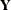, predictors or independent variables, and a linear expectation model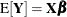relating the two, a primary analytical goal is to estimate or test for the significance of certain linear combinations of the elements of. For least squares regression and analysis of variance, this is accomplished by computing linear combinations of the observeds. An unbiased linear estimate of a specific linear function of the individuals, say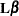, is a linear combination of thes that has an expected value of. Hence, the following definition:

A linear combination of the parametersis estimable if and only if a linear combination of thes exists that has expected value.

Any linear combination of thes, for instance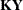, will have expectation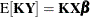. Thus, the expected value of any linear combination of thes is equal to that same linear combination of the rows ofmultiplied by. Therefore,is estimable if and only if there is a linear combination of the rows ofthat is equal to
—that is, if and only if there is a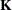such that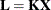.

Thus, the rows ofform a generating set from which any estimablecan be constructed. Since the row space ofis the same as the row space of, the rows ofalso form a generating set from which all estimables can be constructed. Similarly, the rows of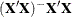also form a generating set for.

Therefore, ifcan be written as a linear combination of the rows of,, or, thenis estimable.

In the context of least squares regression and analysis of variance, an estimable linear function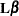can be estimated by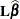, where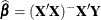. From the general theory of linear models, the unbiased estimatoris, in fact, the best linear unbiased estimator of, in the sense of having minimum variance as well as maximum likelihood when the residuals are normal. To test the hypothesis that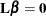, compute the sum of squares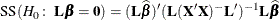and form an F test with the appropriate error term. Note that in contexts more general than least squares regression (for example, generalized and/or mixed linear models), linear hypotheses are often tested by analogous sums of squares of the estimated linear parameters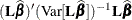.